# Answer the Detail : What Are the Types of Price Elasticity of Demand? - Economics

Short Note

What are the types of Price Elasticity of Demand?

#### Solution 1

The following are the different types of price elasticity of demand:

Perfectly inelastic demand
Demand is said to be perfectly inelastic when the quantity demanded is independent of the price of the good.  In other words, the quantity demanded does not show any responsiveness to the change in the price. Thus, elasticity of demand is perfectly inelastic i.e. |Ed| = 0. This can be represented as follows: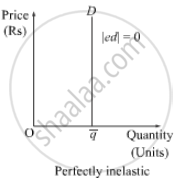Unitary elastic demand
When the demand for a good responds exactly in the same amount as the change in its price, the demand is said to be unitary elastic.  In this case, the percentage change in the demand for a good is equal to the percentage change in its price and |ed| =1.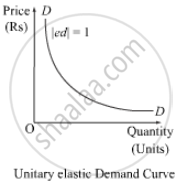Perfectly elastic demand
The demand is said to be perfectly elastic when the price is independent of the quantity demanded. In other words, a slight change in the price can lead to very high change in the quantity demanded. Thus, the elasticity of demand is perfectly elastic i.e. |Ed| = ∞.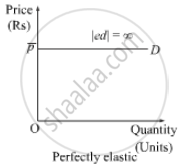Relatively elastic demand
The demand is said to be relatively elastic if it is highly responsive to its price. In this case, the percentage change in demand is greater than the percentage change in the price. Here, |ed| > 1.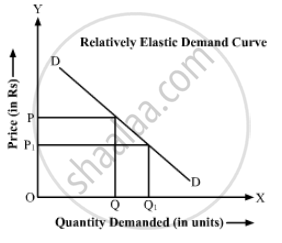Relatively inelastic demand

The demand for a commodity is said to be relatively inelastic when change in price leads to a proportionately less change in the quantity demanded. Here, |ed| < 1.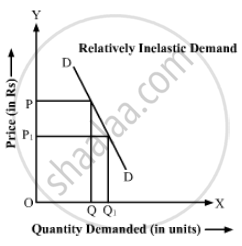The elasticity of demand is less than one i.e. |Ed| < 1.

#### Solution 2

The following are the different types of price elasticity of demand:

Perfectly inelastic demand
Demand is said to be perfectly inelastic when the quantity demanded is independent of the price of the good.  In other words, the quantity demanded does not show any responsiveness to the change in the price. Thus, elasticity of demand is perfectly inelastic i.e. |Ed| = 0. This can be represented as follows:Unitary elastic demand
When the demand for a good responds exactly in the same amount as the change in its price, the demand is said to be unitary elastic.  In this case, the percentage change in the demand for a good is equal to the percentage change in its price and |ed| =1.Perfectly elastic demand
The demand is said to be perfectly elastic when the price is independent of the quantity demanded. In other words, a slight change in the price can lead to very high change in the quantity demanded. Thus, the elasticity of demand is perfectly elastic i.e. |Ed| = ∞.Relatively elastic demand
The demand is said to be relatively elastic if it is highly responsive to its price. In this case, the percentage change in demand is greater than the percentage change in the price. Here, |ed| > 1.Relatively inelastic demand

The demand for a commodity is said to be relatively inelastic when change in price leads to a proportionately less change in the quantity demanded. Here, |ed| < 1.The elasticity of demand is less than one i.e. |Ed| < 1.

Concept: Importance of Price Elasticity of Demand
Is there an error in this question or solution?

#### APPEARS IN

Micheal Vaz Economics HSC 12th Standard Maharashtra State Board
Chapter 4 Elasticity of Demand
Exercise | Q 13 | Page 32
Micheal Vaz Economics HSC 12th Standard Maharashtra State Board
Chapter 4 Elasticity of Demand
Exercise | Q 2 | Page 32